# Calculus 1 : How to find decreasing intervals by graphing functions

## Example Questions

1 3 Next →

### Example Question #21 : Decreasing Intervals

Determine the intervals on which the function is decreasing: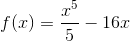The function is never decreasing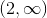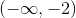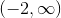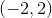Explanation:

To determine the intervals on which the function is decreasing, we must find the intervals on which the first derivative is negative.

The first derivative of the function is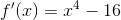and was found using the following rule: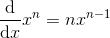Now, we must find the critical values, at which the first derivative is equal to zero: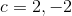Using the critical values, we can make our intervals: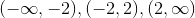Note that at the bounds of the intervals the first derivative is neither positive nor negative.

To determine the sign of the first derivative, simply plug in any point on the interval into the first derivative function: on the first interval, the first derivative is positive, on the second interval it is negative, and on the third interval it is positive. Thus, our answer is.

### Example Question #22 : Decreasing Intervals

Find the decreasing interval of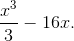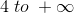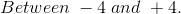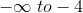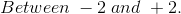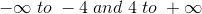Explanation:

We need to set the derivative equal to zero.  Then, we can simply input values to the left and right of the roots to see their sign.  If the output is negative, the function is increasing on that range.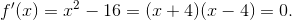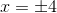The derivative is negative only in the region between these roots.  Therefore, the initial function is decreasing only in between these two values.

### Example Question #23 : Decreasing Intervals

Find the intervals on which the function is decreasing: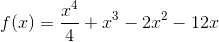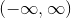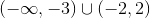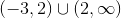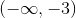Explanation:

To find the intervals on which the function is decreasing, we must find the intervals on which the first derivative of the function is negative.

To start, we must find the first derivative: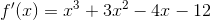The derivative was found using the following rule:Next, we must find the critical values, the values at which the first derivative is equal to zero: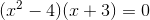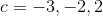Note that the first derivative was simplified using a factoring by grouping.

With the critical values, we can now make our intervals: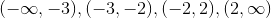Note that at the endpoints of the intervals the first derivative is neither positive nor negative.

To check the sign of the first derivative on the intervals, simply plug in any point on each interval into the first derivative function and see the sign. On the first interval, the first derivative is negative, on the second, it is positive, on the third, it is negative, and on the fourth, it is positive. Thus, the intervals on which the function is decreasing are.

### Example Question #24 : Decreasing Intervals

Determine the intervals on which the function is decreasing: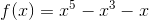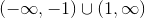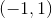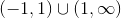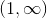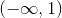Explanation:

To find the intervals on which the function is decreasing, we must find the intervals on which the first derivative of the function is negative.

The first derivative of the function is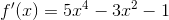and was found using the following rule:Next, we must find the critical values, or values at which the first derivative of the function is equal to zero: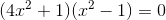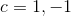Now, using the critical values, we can create the intervals: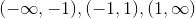Note that at the endpoints of the intervals, the first derivative is neither positive nor negative.

To determine the sign of the first derivative on each interval, simply plug in any value on each interval into the first derivative function and check the sign. On the first interval, the first derivative is positive, on the second interval, it is negative, and on the third, it is positive. Thus, the interval on which the function is decreasing is.

### Example Question #25 : Decreasing Intervals

Is the function g(x) increasing or decreasing on the interval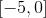?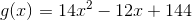Decreasing, because g'(x) is negative on the given interval.

Increasing, because g'(x) is negative on the given interval.

Increasing, because g'(x) is positive on the given interval.

Decreasing, because g'(x) is positive on the given interval.

Decreasing, because g'(x) is negative on the given interval.

Explanation:

Is the function g(x) increasing or decreasing on the interval?To tell if a function is increasing or decreasing, we need to see if its first derivative is positive or negative. let's find g'(x)

Recall that the derivative of a polynomial can be found by taking each term, multiplying by its exponent and then decreasing the exponent by 1.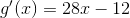Next, we need to see if the function is positive or negative over the given interval.

Begin by finding g'(-5)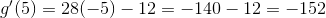So, g'(-5) is negative, but what about g'(0)?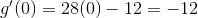Also negative, so our answer is:

Decreasing, because g'(x) is negative on the interval.

1 3 Next →